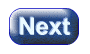created 08/19/97; revised 08/01/00; 09/14/00; 07/19/03

# CHAPTER 1 — Vectors, Points, and Column Matrices

This chapter discusses the objects of computer graphics—vectors and points—and how they are represented in a computer—as column matrices. A column matrix is a mathematical object that has many uses besides its use in computer graphics. These notes discuss only how they are used in computer graphics.

#### Chapter Topics:

• Computer graphics as modeling and viewing.
• Geometrical points and vectors.
• Column and row matrices.
• Calculating displacements.
• Equality of column matrices.
• Names for column matrices.
• Representing points with column matrices.

Geometical points and vectors are essential in computer graphics. They must be represented in a way that is easy to manipulate both on paper and with a program. A column matix is the usual choice for this.

Some graphics books use the term column vector for the object that these notes call a column matrix. This is just a variation in terminology and does not affect the concepts or formulas presented here.

A worse problem is that some books use row matrices. Column matrices and row matrices are equivalent, but equations written using row matrices are not the same as those written using column matrices. The differences are easy to adjust for, but annoying.

### QUESTION 1:(Review: ) What two types of geometrical objects are represented with column matrices?